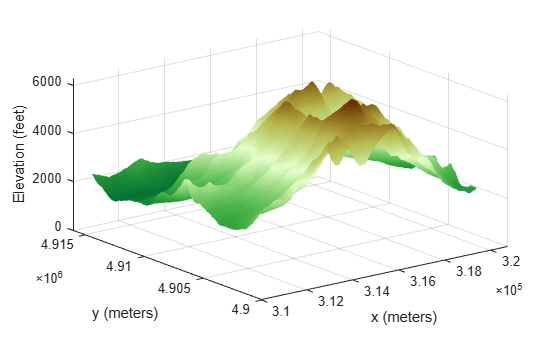# worldGrid

World coordinates of raster elements

## Syntax

``[X,Y] = worldGrid(R)``
``[X,Y] = worldGrid(R,gridOption)``

## Description

example

````[X,Y] = worldGrid(R)` returns the world coordinates of raster elements as the 2-D arrays `X` and `Y`. The coordinates of raster element `(i,j)` are `(X(i,j),Y(i,j))`.```

example

````[X,Y] = worldGrid(R,gridOption)`, where `gridOption` is `'gridvectors'`, returns `X` and `Y` as row vectors. The coordinates of raster element `(i,j)` are `(X(j),Y(i))`. The default for `gridOption` is `'fullgrid'`, which returns `X` and `Y` as 2-D arrays.```

## Examples

collapse all

Import elevation data, find the coordinates of the imported data, then display the data as a surface.

First import elevation data  for an area around Mount Washington as an array and a map cells reference object. Prepare the data for plotting by replacing missing data values with `0`. Then, find the coordinates of each element in the array.

```[Z,R] = readgeoraster('MtWashington-ft.grd','OutputType','double'); info = georasterinfo('MtWashington-ft.grd'); Z = standardizeMissing(Z,info.MissingDataIndicator); [X,Y] = worldGrid(R);```

Display the elevation data as a surface using an appropriate colormap. Set the `EdgeColor` property to `'none'` to remove the mesh from the surface.

```surf(X,Y,Z,'EdgeColor','none') demcmap(Z) xlabel('x (meters)') ylabel('y (meters)') zlabel('Elevation (feet)')```The elevation data used in this example is courtesy of the U.S. Geological Survey.

Create a map cells reference object for a 3-by-4 raster with x values in the range [7000, 7400] meters and y values in the range [2700, 3300] meters. Get the coordinates of the raster elements and return them as row vectors.

```R = maprefcells([7000 7400],[2700 3300],[3 4]); [X,Y] = worldGrid(R,'gridvectors')```
```X = 1×4 7050 7150 7250 7350 ```
```Y = 1×3 2800 3000 3200 ```

If you do not specify the second argument as `'gridvectors'`, then the `worldGrid` function returns 2-D arrays by default.

`[xFull,yFull] = worldGrid(R)`
```xFull = 3×4 7050 7150 7250 7350 7050 7150 7250 7350 7050 7150 7250 7350 ```
```yFull = 3×4 2800 2800 2800 2800 3000 3000 3000 3000 3200 3200 3200 3200 ```

## Input Arguments

collapse all

Spatial reference, specified as a `MapCellsReference` or `MapPostingsReference` object.

If `R` is a `MapCellsReference` object, then `X` and `Y` are cell centers. If `R` is a `MapPostingsReference` object, then `X` and `Y` are posting points.

Grid option, specified as one of these values:

• `'fullgrid'` — Return `X` and `Y` as 2-D arrays, where each row of `X` is identical and each column of `Y` is identical. This is the default behavior.

• `'gridvectors'` — Return `X` and `Y` as row vectors. Use this option when you want to reduce memory usage and when 2-D arrays are unnecessary, such as when plotting large data sets with the `surf` function. You can specify `gridOption` as `'gridvectors'` only when the `TransformationType` property of `R` has a value of `'rectilinear'`.

This table shows the difference between `'fullgrid'` and `'gridvectors'`.

`'fullgrid'``'gridvectors'`
```R = maprefcells([7000 7400],[2700 3300],[3 4]); [X,Y] = worldGrid(R)```
```X = 7050 7150 7250 7350 7050 7150 7250 7350 7050 7150 7250 7350 Y = 2800 2800 2800 2800 3000 3000 3000 3000 3200 3200 3200 3200```
```R = maprefcells([7000 7400],[2700 3300],[3 4]); [X,Y] = worldGrid(R,'gridvectors')```
```X = 7050 7150 7250 7350 Y = 2800 3000 3200 ```

Data Types: `char` | `string`

## Output Arguments

collapse all

x-coordinates, returned as a 2-D array or a row vector. By default, `X` is a 2-D array. To return `X` as a row vector, specify `gridOption` as `'gridvectors'`.

By default, and when `gridOption` is `'fullgrid'`, the sizes of `X` and `Y` each equal the `RasterSize` property of `R`. When `gridOption` is `'gridvectors'`, the lengths of `X` and `Y` equal the second and first elements of the `RasterSize` property of `R`, respectively.

y-coordinates, returned as a 2-D array or a row vector. By default, `Y` is a 2-D array. To return `Y` as a row vector, specify `gridOption` as `'gridvectors'`.

By default, and when `gridOption` is `'fullgrid'`, the sizes of `X` and `Y` each equal the `RasterSize` property of `R`. When `gridOption` is `'gridvectors'`, the lengths of `X` and `Y` equal the second and first elements of the `RasterSize` property of `R`, respectively.

## Version History

Introduced in R2021a